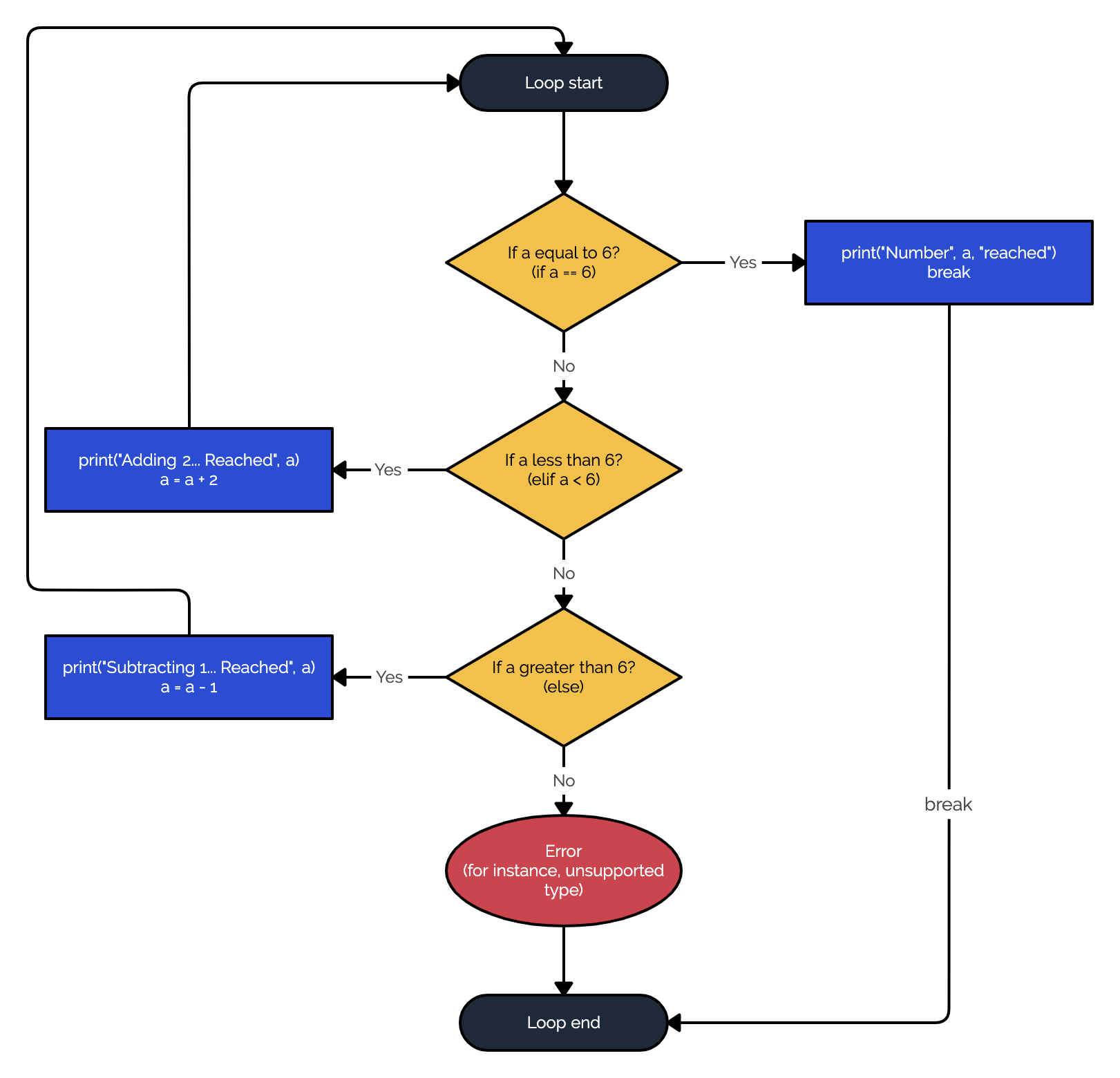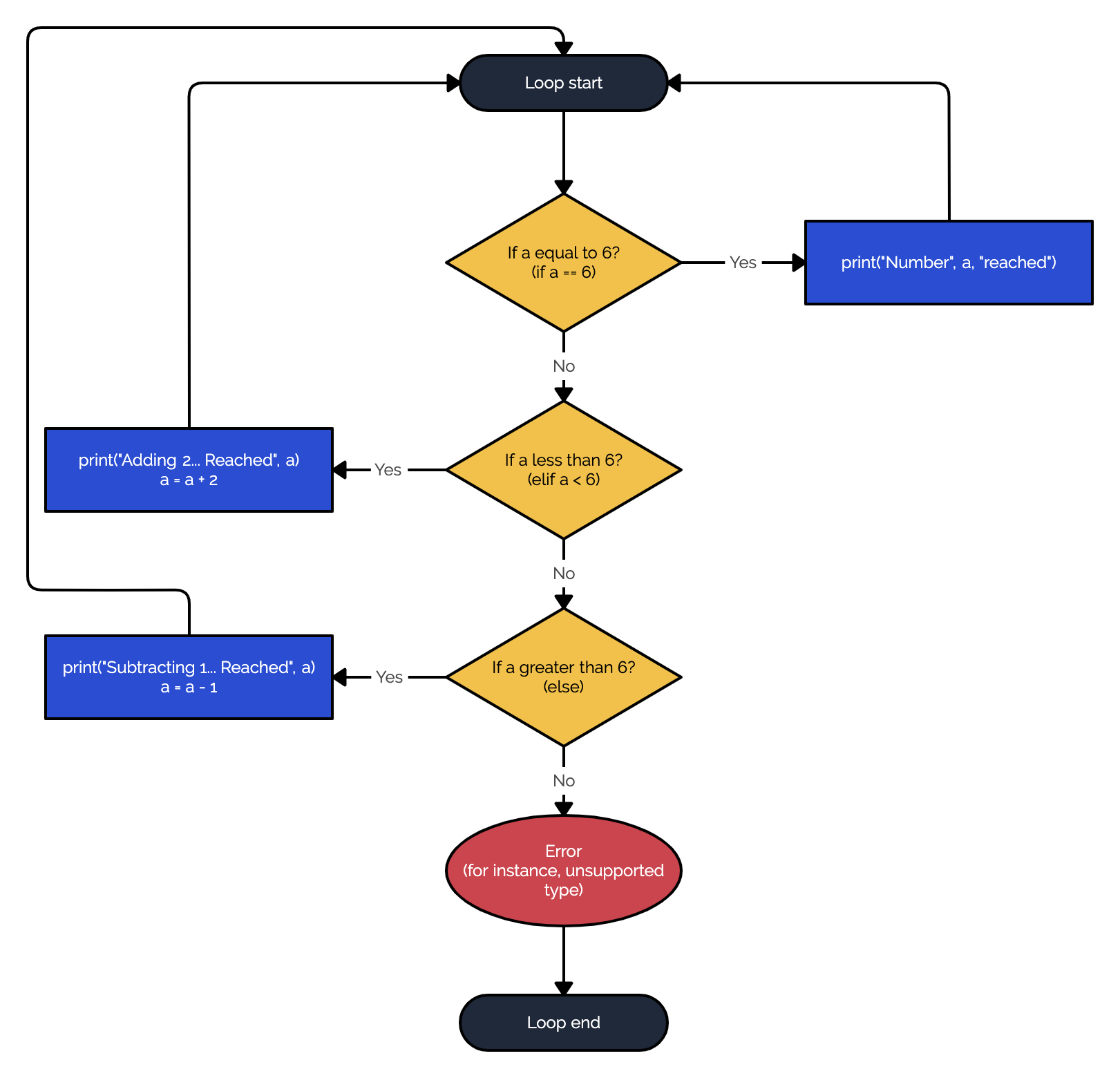Course Content

Introduction to Pythonwhile True

Sometimes we may not know when to stop the loop execution. In this case, we can launch an infinite loop, and then within the loop body, we set the 'stopping point'.

To launch an infinite loop, use `while True` syntax. To stop the loop execution, use the `break` keyword. If at some point you need to skip the rest of the code within the loop body and start again, you need to use the `continue` keyword.

For instance, assume that we have number 3 and want to reach number 6. All we can do is add 2 and subtract 1.The loop above can be visualized as follows:If we don't specify `break` in the first `if`, then we'll get an infinite loop because 6 is not satisfying any other condition but the first one. In that case, it prints us "Number 6 reached" an infinite number of times.As you can see above, without the `break` keyword, all the actions will lead to the loop start.

Section 5.

Chapter 2